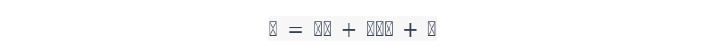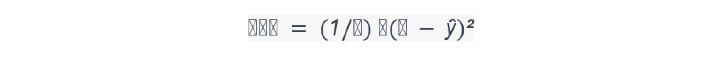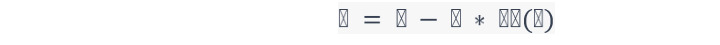# Gradient Descent in Linear Regression

The use of linear regression is a useful technique for figuring out and examining the relationship between variables. Predictive modeling relies on it and uses it as the cornerstone for many machine learning techniques. Machine learning requires a lot of optimization. It is comparable to improving a model to provide it with the best performance. Gradient descent, a key technique in optimization, enters the picture at this point. Consider it as a trustworthy ally that will help us navigate the vast array of potential model parameters to find the optimal ones.

Gradient descent allows us to iteratively change these parameters by moving slightly in the direction of the steepest fall, minimizing the error over time, and bringing about convergence. Gradient descent is an essential technique in machine learning since it allows us to efficiently optimize the performance of linear regression models. In this post, we will look closely at Gradient Descent in Linear Regression.

## Understanding Linear Regression

An important statistical method for simulating the connection between a dependent variable and one or more independent variables is linear regression. Finding the appropriate line to depict the connection between the variables entails fitting a linear equation to a pre−existing dataset. A simple linear regression equation is stated as:Where

• The dependent variable is y.

• The independent variable is x.

• y−intercept (the value of y when x is 0) is $\mathrm{\beta_{0}}$

• Slope (the change in y for a one−unit increase in x) is $\mathrm{\beta_{1}}$

• The random error is represented by $\mathrm{\varepsilon}$

The goal of linear regression is to minimize the difference between the predicted values () and the actual values (y), often known as cost, loss, or error. The mean squared error (MSE), which is outlined as follows, is the objective function that is most frequently utilized.Where,

• The total number of observations is n.

• y is the dependent variable's actual value.

• ŷ is the dependent variable's anticipated value.

The cost function in machine learning models is iteratively minimized using the potent optimization process known as gradient descent. It is a commonly used method in several fields, including linear regression. In a nutshell, gradient descent converges to the best solution by repeatedly modifying the model parameters in the direction of the cost function's steepest fall.

Gradient descent can be represented mathematically as follows:Here, the model parameters are represented by $\mathrm{\theta}$, the learning rate is indicated by $\mathrm{\alpha}$, the cost function's gradient with respect to the parameters is denoted by $\mathrm{\bigtriangledown J(\theta)}$, and the multiplication operation is shown by *. The model gets closer to the ideal parameter values that minimize the cost function as it updates the parameters in accordance with this formula.

Finding the best−fit line in the context of linear regression that minimizes the gap between the predicted and actual values depends critically on gradient descent. The mean squared error (MSE) is frequently used as the cost function in linear regression. Gradient descent allows the model to modify its predictions, lowering the overall error and increasing the precision of the regression line. This is done by iteratively updating the parameters (slope and intercept) using the gradient of the MSE.

### Implementing Gradient Descent in Linear Regression

An example of functional Python code that uses gradient descent to do linear regression is shown below:

import numpy as np
import matplotlib.pyplot as plt

# Generate sample data for demonstration
np.random.seed(42)
X = 2 * np.random.rand(100, 1)
y = 4 + 3 * X + np.random.randn(100, 1)

# Add bias term to X
X_b = np.c_[np.ones((100, 1)), X]

# Set hyperparameters
learning_rate = 0.01
num_iterations = 1000

# Initialize parameters randomly
theta = np.random.randn(2, 1)

for iteration in range(num_iterations):
gradients = 2 / 100 * X_b.T.dot(X_b.dot(theta) - y)
theta = theta - learning_rate * gradients

# Print the final parameter values
print("Intercept:", theta)
print("Slope:", theta)

# Plot the data points and the regression line
plt.scatter(X, y)
plt.plot(X, X_b.dot(theta), color='red')
plt.xlabel('X')
plt.ylabel('y')
plt.show()


### Output

Intercept: 4.158093763822134
Slope: 2.8204434017416244We begin this code by producing some example data. The bias term is subsequently included in the input matrix X to account for the intercept in the linear regression equation. The gradient descent algorithm's learning rate and iterations are then set. Gradient descent is then performed by iterating through the predetermined number of iterations after randomly initializing theta parameters. We compute the gradients using the mean squared error loss function in each iteration and adjust the parameters appropriately. Finally, we use Matplotlib to print the final intercept and slope values and display the data points and fitted regression lines.

## Conclusion

The effects of linear regression go well beyond the realm of predictive modeling. Linear regression enables data−driven decision−making across a variety of disciplines by revealing the correlations between variables. Organizations can acquire insights into consumer behavior, optimize resource allocation, estimate demand, and make wise strategic decisions using linear regression in industries including finance, economics, marketing, and healthcare.

Updated on: 24-Aug-2023

125 Views